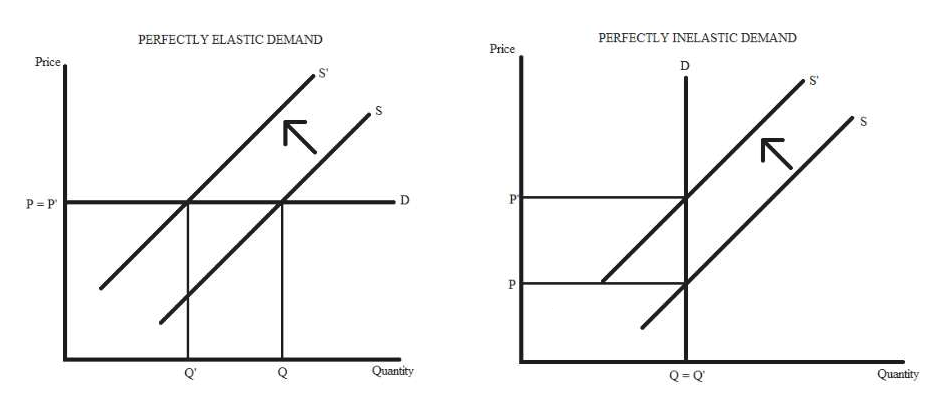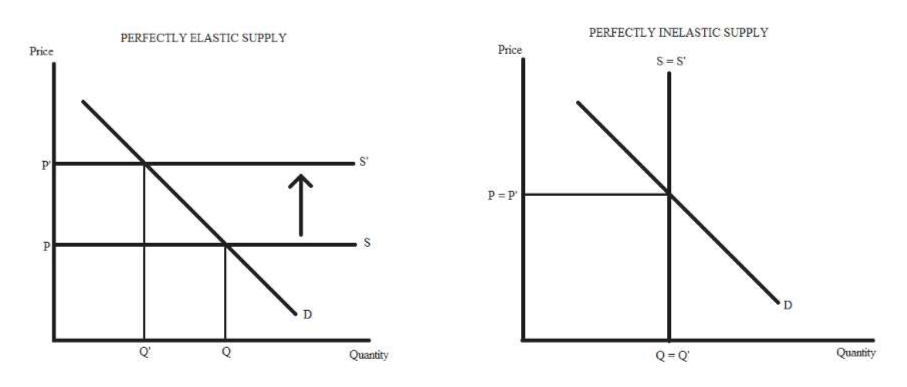# Taxes or cost increases are not always paid where they are laid. how to use examples to explain the concept of the incidence of a tax or extra cost burden.

Question

Taxes or cost increases are not always paid where they are laid. how to use examples to explain the concept of the incidence of a tax or extra cost burden.

check_circleExpert Solution
Step 1

Yes, it is true that taxes are not always paid where they are laid as the burden of tax depends on the price elasticity of demand and supply.

Step 2

There are two cases when the demand is perfectly elastic and when the demand is perfectly inelastic.

In the first case where the demand is perfectly elastic, an imposition of tax shifts the supply curve to the left and there is no change in the equilibrium price. So, the entire tax burden is born by the sellers.

In the second case, the demand is perfectly inelastic i.e. buyers will demand the same quantity irrespective of the price. A tax imposed by the government shifts the supply curve upwards and the equilibrium price rises by the same amount. So, the tax burden is borned by the buyers.help_outlineImage TranscriptionclosePERFECTLY INELASTIC DEMAND PERFECTLY ELASTIC DEMAND Price Price S D P P Quantity Q Q Quantity fullscreen
Step 3

There are two cases: The first case shows the perfectly elastic supply and the second case shows the perfectly inelastic supply.

In the first case, the supply curve moves from S to S' due to the imposition of tax by th...help_outlineImage TranscriptionclosePERFECTLY INELASTIC SUPPLY PERFECTLY ELASTIC SUPPLY Price Price S-S P = P D Q Q Q Quantity Quantity in D fullscreen

### Want to see the full answer?

See Solution

#### Want to see this answer and more?

Solutions are written by subject experts who are available 24/7. Questions are typically answered within 1 hour*

See Solution
*Response times may vary by subject and question
Tagged in